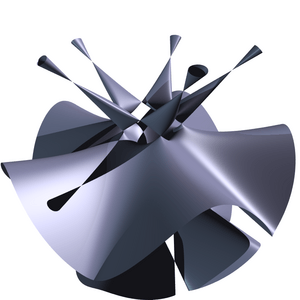# Algebraic geometry facts for kids

Kids Encyclopedia FactsThis Togliatti surface is an algebraic surface of degree five. The picture represents a portion of its real locus

Algebraic geometry is a branch of mathematics studying polynomial equations. Modern algebraic geometry is based on more abstract techniques of abstract algebra, especially commutative algebra, with the language and the problems of geometry.

The main objects of study in algebraic geometry are algebraic varieties, which are geometric manifestations of sets of solutions of systems of polynomial equations. Examples of the most studied classes of algebraic varieties are: plane algebraic curves, which include lines, circles, parabolas, ellipses, hyperbolas, cubic curves like elliptic curves and quartic curves like lemniscates, and Cassini ovals. A point of the plane belongs to an algebraic curve if its coordinates satisfy a given polynomial equation. Basic questions involve the study of the points of special interest like the singular points, the inflection points and the points at infinity. More advanced questions involve the topology of the curve and relations between the curves given by different equations.

Algebraic geometry occupies a central place in modern mathematics. The concepts it uses connects it to such diverse fields as complex analysis, topology and number theory. At the start, algebraic geometry was about studying systems of polynomial equations in several variables. Algebraic geometry starts at the point where equation solving leaves off: In many cases, finding the properties all the solutions a given set of equations have is more important than finding a particular solution: this leads into some of the deepest areas in all of mathematics, both conceptually and in terms of technique.

In the 20th century, algebraic geometry has split into several subareas.

• The main stream of algebraic geometry is devoted to the study of the complex points of the algebraic varieties and more generally to the points with coordinates in an algebraically closed field.
• The study of the points of an algebraic variety with coordinates in the field of the rational numbers or in a number field became arithmetic geometry (or more classically Diophantine geometry), a subfield of algebraic number theory.
• The study of the real points of an algebraic variety is the subject of real algebraic geometry.
• A large part of singularity theory is devoted to the singularities of algebraic varieties.
• When computers became more common, a field named 'computational algebraic geomery' developed. It looks at intersection of algebraic geometry and computer algebra. It is concerned with the development of algorithms and software for studying and finding the properties of explicitly given algebraic varieties.

Much of the development of the main stream of algebraic geometry in the 20th century occurred within an abstract algebraic framework, with increasing emphasis being placed on "intrinsic" properties of algebraic varieties not dependent on any particular way of embedding the variety in an ambient coordinate space. The developments in topology, differential and complex geometry occurred much in the same way. One key achievement of this abstract algebraic geometry is Grothendieck's scheme theory which allows one to use sheaf theory to study algebraic varieties in a way which is very similar to its use in the study of differential and analytic manifolds. This is obtained by extending the notion of point: In classical algebraic geometry, a point of an affine variety may be identified, through Hilbert's Nullstellensatz, with a maximal ideal of the coordinate ring, while the points of the corresponding affine scheme are all prime ideals of this ring. This means that a point of such a scheme may be either a usual point or a subvariety. This approach also enables a unification of the language and the tools of classical algebraic geometry, mainly concerned with complex points, and of algebraic number theory. Wiles's proof of the longstanding conjecture called Fermat's last theorem is an example of the power of this approach.

## Images for kidsIn Spanish: Geometría algebraica para niños